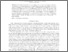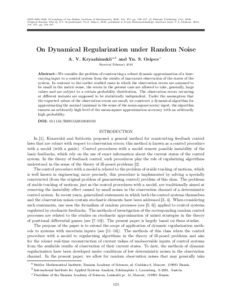On dynamical regularization under random noise

Kryazhimskiy, A.V. & Osipov, Y.S. (2010). On dynamical regularization under random noise. Proceedings of the Steklov Institute of Mathematics 271 (1) 125-137. 10.1134/S0081543810040103.Preview
Text
XJ-10-154.pdf

Abstract

We consider the problem of constructing a robust dynamic approximation of a time-varying input to a control system from the results of inaccurate observation of the states of the system. In contrast to the earlier studied cases in which the observation errors are assumed to be small in the metric sense, the errors in the present case are allowed to take, generally, large values and are subject to a certain probability distribution. The observation errors occurring at different instants are supposed to be statistically independent. Under the assumption that the expected values of the observation errors are small, we construct a dynamical algorithm for approximating the normal (minimal in the sense of the mean-square norm) input; the algorithm ensures an arbitrarily high level of the mean-square approximation accuracy with an arbitrarily high probability.

Item Type: Article Dynamic Systems (DYN) Proceedings of the Steklov Institute of Mathematics; 271(1):125-137 (December 2010) IIASA Import 15 Jan 2016 08:43 27 Aug 2021 17:21 http://pure.iiasa.ac.at/9164View Item